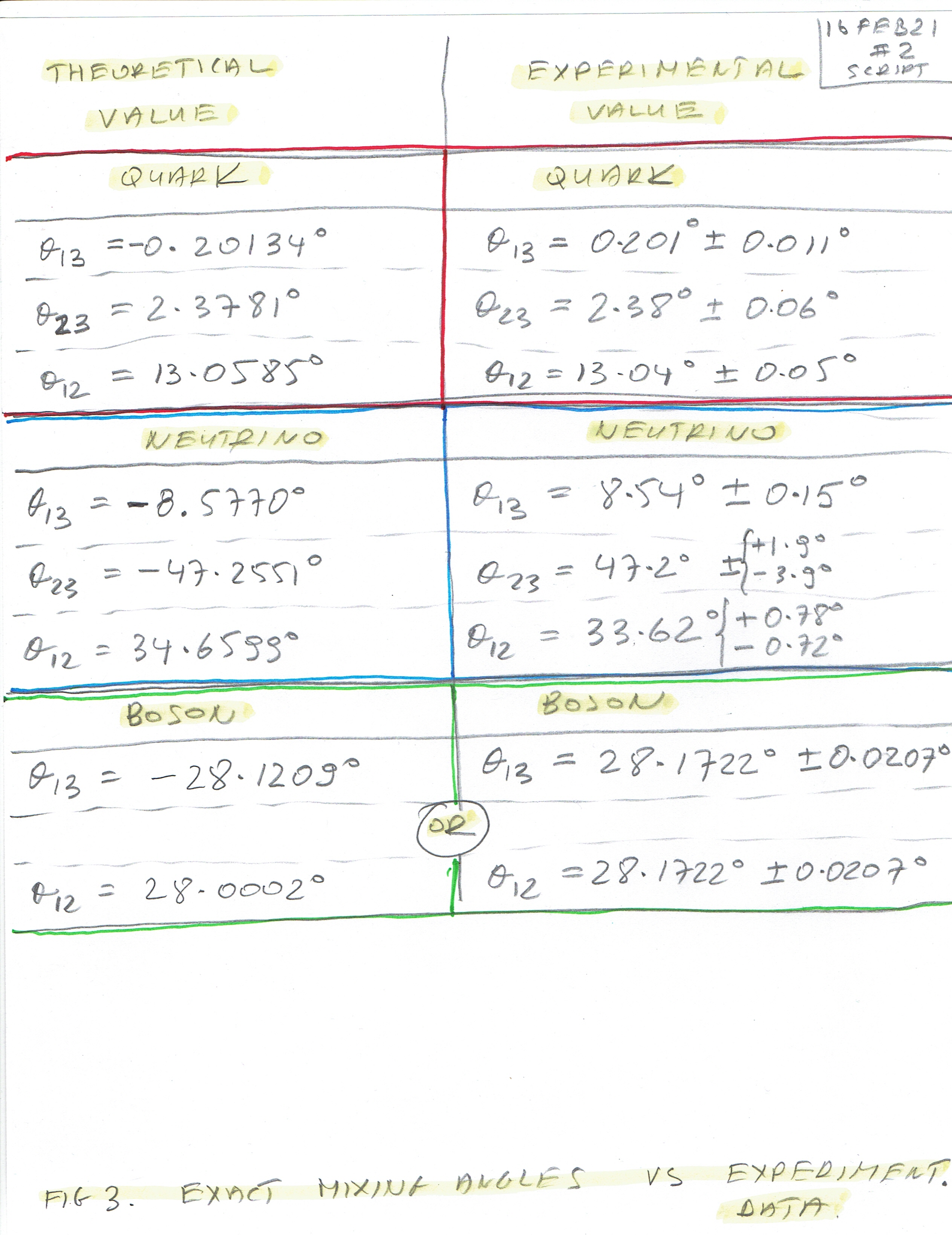17 February 2021 AD

Ash Wednesday; Seven Holy Founders of the Order of Servites (1233)

Apart from the Four Fundamental Coupling Constants of the four fundamental forces (where only fine structure constant alpha is calculated well), there are also seven mixing (oscillation) angles known (quark, neutrino and one of boson) and two CP Violating Phase Angles (quark and neutrino). So, altogether we have ten known parameters.

This may seem not enough to predict all the other missing parameters of the remaining elements - but in fact, it is quite enough. One can deduce remaining ones from those ten parameters, especially that that there are some "educated guesses" as to coupling constants for example.

This article in the form of a script, shows theoretical and experimental values of Mixing (Oscillation) Angles (theta) of Quark, Neutrino (three each) and one of a Boson (Weinberg Angle).

By way of deduction, all missing ones are calculated as well - (two thetas of Boson) and three thetas for the Graviton.

In "Figure 1 - Mixing (Oscillation) Angles θINITIAL (i.e. the experimental mixing angles) and θFINAL (i.e. the same angles after the transformation)"  you can see values of the mixing angles for all four elements. I insist that those angles are calculated well - there will be two tests at the end of this series showing clearly that those are correct numbers indeed. And in future works, I will show (probably - Deus Vult) other proofs. Anyway, it is possible to have different values of the angles for Boson and Graviton, but they must add to the same number as do those I have calculated - it will be proven in the future once the equations of mass are being used.

"Figure 1 - Mixing (Oscillation) Angles θINITIAL (i.e. the experimental mixing angles) and θFINAL (i.e. the same angles after the transformation)":

Attention: C0, C1, C2, C3, C4, C5 and CM1, CM2, CM3, CM4, CM5, CM6 stand for the constants from which the particular angles were calculated.In the future articles, I will show the printouts from the programs (and possibly FORTRAN programs as well) so you know better from where those values were obtained.

The second jpg file shows how to calculate Weinberg Angle.

"Figure 2 - Weinberg Angle - Mixing (Oscillation) Angle of a Boson":The third jpg shows theoretical and experimental values of Quark, Neutrino (three angles each) and a Boson (one entry).

Figure 3 - Exact Mixing (Oscillation) Angles vs Experimental Data: